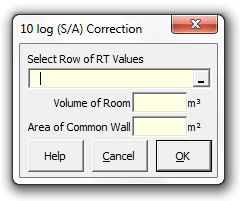### Strutt Help

10 log (S/A) Correction

Strutt|Transmission Loss|10log(S/A) Correction inserts the 10log(S/A) correction into the active row of the worksheet, where S (m²) is the surface area of the common wall between two rooms and A is the absorption area (m² sabin) of the receiving room.The correction uses the following formula (re-arranged Sabine RT formula) to calculate the absorption area A of the receiving room given the room volume V(m³) and the Reverberation Times RT (s) of the room:

A = (0.161V)/(RT)